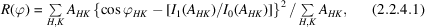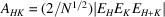International
Tables for
Crystallography
Volume F
Crystallography of biological macromolecules
Edited by E. Arnold, D. M. Himmel and M. G. Rossmann

International Tables for Crystallography (2012). Vol. F, ch. 2.2, pp. 68-69

## Section 2.2.4. Quality indicators for substructure determination

H. M. Einspahra* and M. S. Weissb

aPO Box 6483, Lawrenceville, NJ 08648–0483, United States, and bHelmholtz-Zentrum Berlin für Materialien und Energie, Macromolecular Crystallography (HZB-MX), Albert-Einstein-Str. 15, D-12489 Berlin, Germany
Correspondence e-mail:  hmeinspahr@yahoo.com

### 2.2.4. Quality indicators for substructure determination

| top | pdf |

The first step of a crystal structure determination after processing and analysing the diffraction data is to use the observed isomorphous and/or anomalous differences in order to determine the isomorphous or anomalous substructure. Since such sub­structures typically consist of rather few atoms, which are relatively far apart, direct-methods approaches have been adopted from small-molecule crystallography and have largely superseded pure Patterson-based methods. The most widely used computer programs for substructure determination are SHELXD (Schneider & Sheldrick, 2002; Sheldrick, 2008), SnB (Weeks et al., 1993, 1994) and HySS (Grosse-Kunstleve & Adams, 2003).

Correlation coefficient CC(all). CC(all) measures the correlation of the E values (analogous to normalized structure factors; Hauptman & Karle, 1953) derived from the observed isomorphous or anomalous differences and those calculated from the substructure model. CC(all) is another application of the Pearson linear correlation coefficient [see equation (2.2.2.13)] and is calculated for all reflections. In the equation, x and y are the E values derived from the observed and calculated differences. CC(all) is typically used to assess the successful determination of the isomorphous or anomalous substructure in a macromolecule. A value of CC(all) ≥ 0.3 often indicates that the substructure has been correctly identified (Schneider & Sheldrick, 2002).

Correlation coefficient CC(weak). Like CC(all), CC(weak) is the Pearson linear correlation coefficient [see equation (2.2.2.13)] between the E values (analogous to normalized structure factors as above) derived from the observed isomorphous or anomalous differences and those calculated from the substructure model. In contrast to CC(all), however, CC(weak) is calculated for the weak reflections only. As above, x and y are the E values derived from the observed and calculated differences. The E-value cutoff for defining a reflection as weak can be chosen by the user, but a typical threshold value is 1.5, although lower values may be required for low-resolution data (Schneider & Sheldrick, 2002). A value of CC(weak) ≥ 0.15 often indicates that the substructure has been correctly identified (Sheldrick, 2010).

The minimal function, R(ϕ). The minimal function R(ϕ) is a measure of the mean-square difference between the values of the triplets calculated using a particular set of phases and the expected values of the same triplets as given by the ratio of modified Bessel functions. The minimal function is expected to have a constrained global minimum when the phases are equal to their correct values for some choice of origin and enantiomorph (the minimal principle).wherewith N being the number of atoms in the corresponding primitive unit cell. The minimal function is the function minimized in the program Shake&Bake, abbreviated as SnB (Weeks et al., 1993, 1994).

### References

Grosse-Kunstleve, R. W. & Adams, P. D. (2003). Substructure search procedures for macromolecular structures. Acta Cryst. D59, 1966–1973.
Hauptman, H. & Karle, J. (1953). Solution of the phase problem. I. The centrosymmetric crystal. Am. Crystallogr. Assoc. Monograph No. 3. Dayton, Ohio: Polycrystal Book Service.
Schneider, T. R. & Sheldrick, G. M. (2002). Substructure solution with SHELXD. Acta Cryst. D58, 1772–1779.
Sheldrick, G. M. (2008). A short history of SHELX. Acta Cryst. A64, 112–122.
Sheldrick, G. M. (2010). Experimental phasing with SHELXC/D/E: combining chain tracing with density modification. Acta Cryst. D66, 479–485.
Weeks, C. M., De Titta, G. T., Miller, R. & Hauptman, H. A. (1993). Application of the minimal principle to peptide structures. Acta Cryst. D49, 179–181.
Weeks, C. M., DeTitta, G. T., Hauptman, H. A., Thuman, P. & Miller, R. (1994). Structure solution by minimal-function phase refinement and Fourier filtering. II. Implementation and applications. Acta Cryst. A50, 210–220.